Скачать презентацию Newton s First Law of Motion Inertia Chapter 4

b673c2ef32b2cf8801f6700ed21b377b.ppt

• Количество слайдов: 18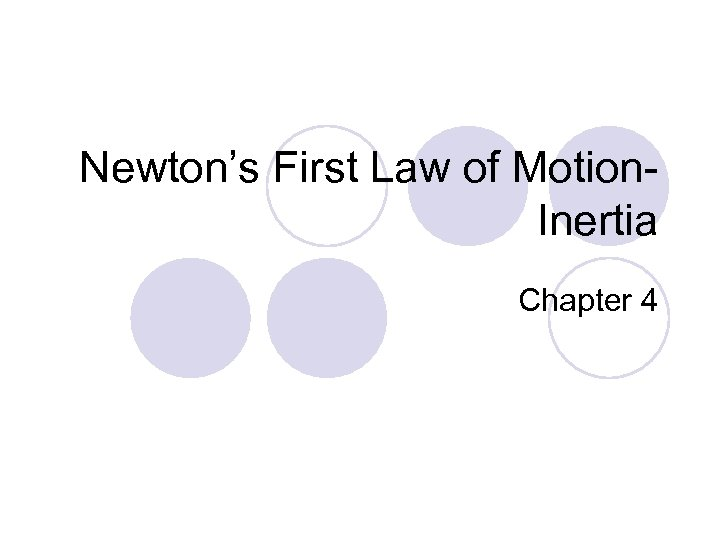Newton’s First Law of Motion. Inertia Chapter 4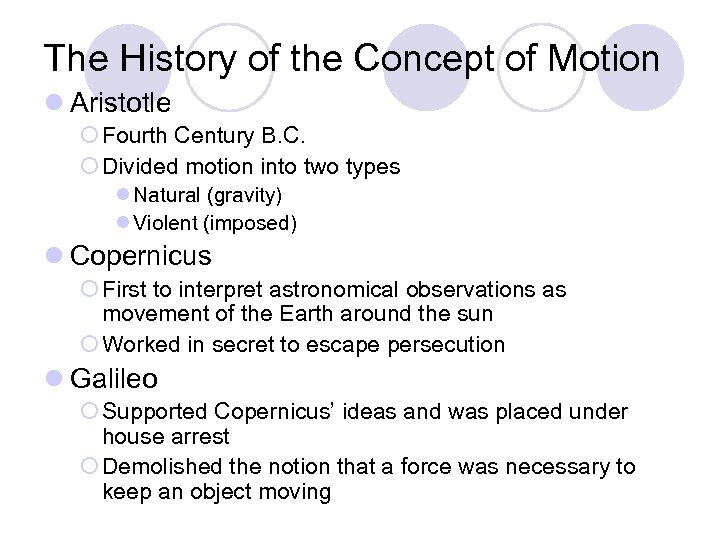The History of the Concept of Motion l Aristotle ¡ Fourth Century B. C. ¡ Divided motion into two types l Natural (gravity) l Violent (imposed) l Copernicus ¡ First to interpret astronomical observations as movement of the Earth around the sun ¡ Worked in secret to escape persecution l Galileo ¡ Supported Copernicus’ ideas and was placed under house arrest ¡ Demolished the notion that a force was necessary to keep an object moving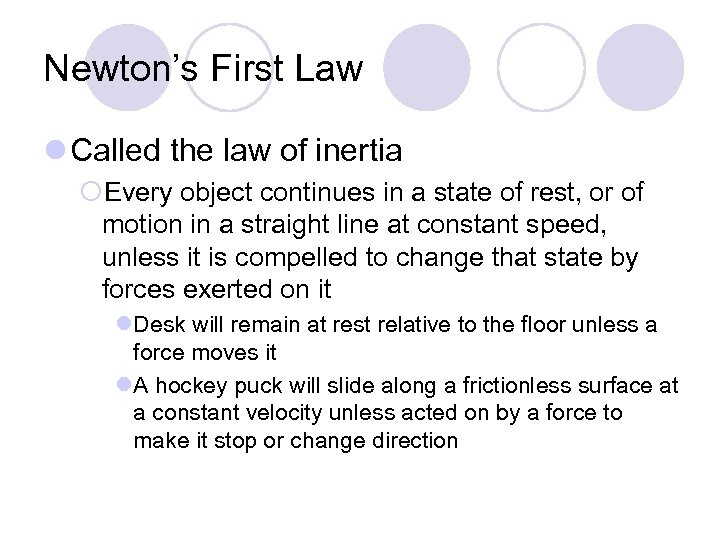Newton’s First Law l Called the law of inertia ¡Every object continues in a state of rest, or of motion in a straight line at constant speed, unless it is compelled to change that state by forces exerted on it l. Desk will remain at rest relative to the floor unless a force moves it l. A hockey puck will slide along a frictionless surface at a constant velocity unless acted on by a force to make it stop or change direction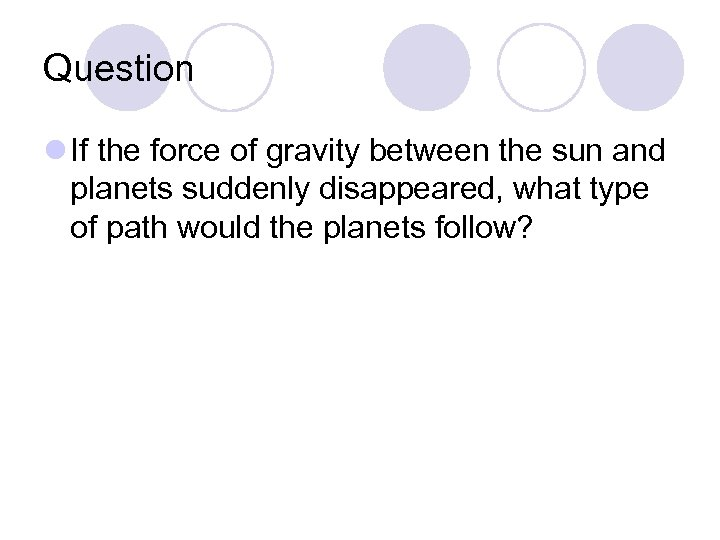Question l If the force of gravity between the sun and planets suddenly disappeared, what type of path would the planets follow?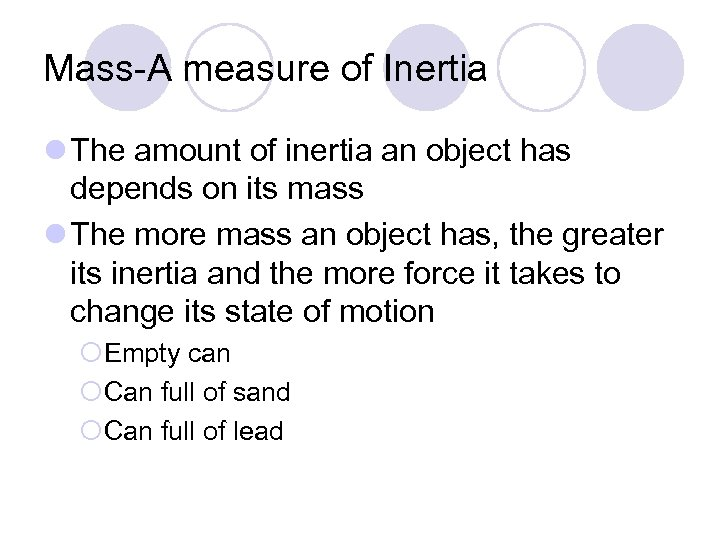Mass-A measure of Inertia l The amount of inertia an object has depends on its mass l The more mass an object has, the greater its inertia and the more force it takes to change its state of motion ¡Empty can ¡Can full of sand ¡Can full of lead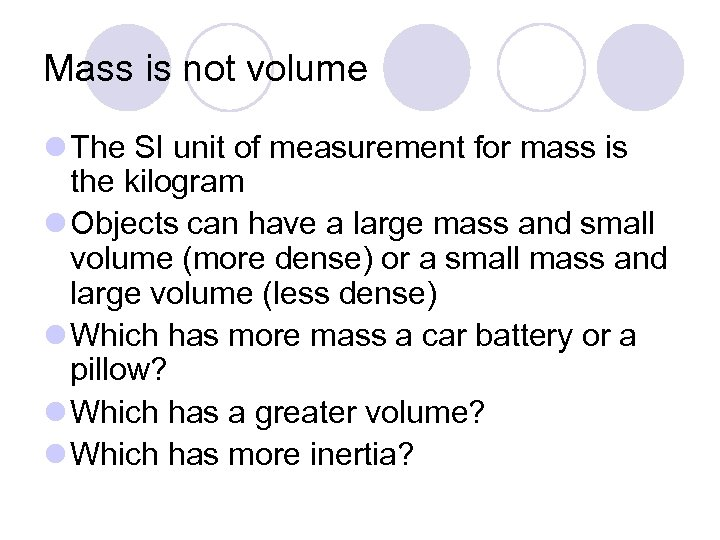Mass is not volume l The SI unit of measurement for mass is the kilogram l Objects can have a large mass and small volume (more dense) or a small mass and large volume (less dense) l Which has more mass a car battery or a pillow? l Which has a greater volume? l Which has more inertia?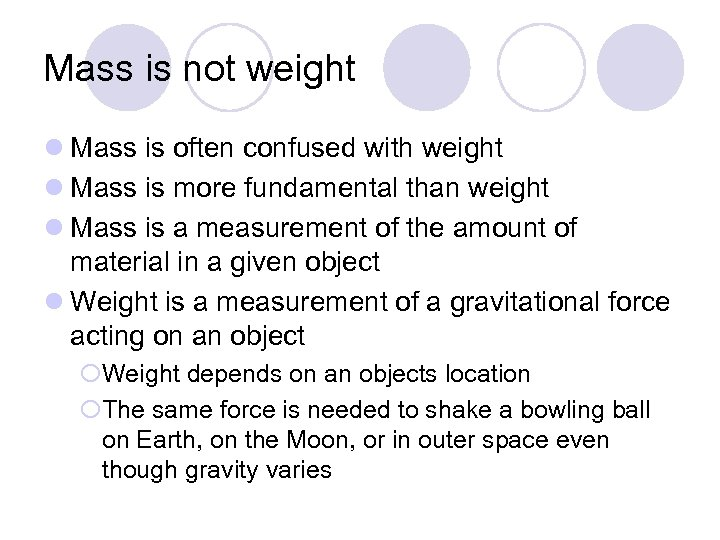Mass is not weight l Mass is often confused with weight l Mass is more fundamental than weight l Mass is a measurement of the amount of material in a given object l Weight is a measurement of a gravitational force acting on an object ¡Weight depends on an objects location ¡The same force is needed to shake a bowling ball on Earth, on the Moon, or in outer space even though gravity varies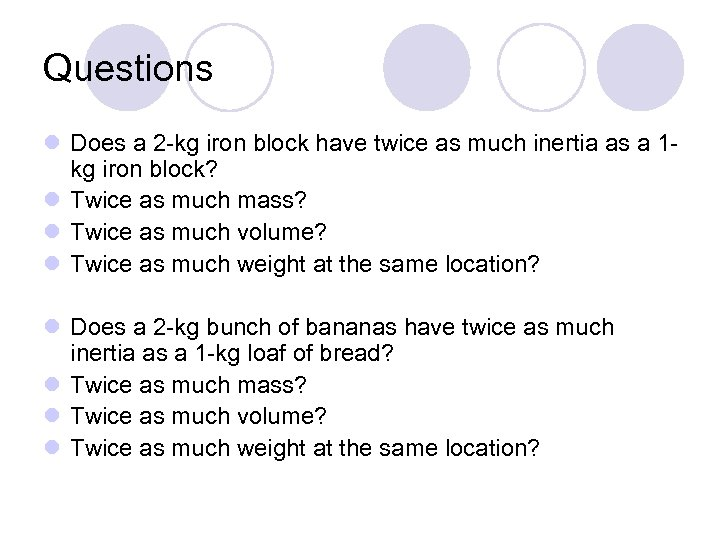Questions l Does a 2 -kg iron block have twice as much inertia as a 1 kg iron block? l Twice as much mass? l Twice as much volume? l Twice as much weight at the same location? l Does a 2 -kg bunch of bananas have twice as much inertia as a 1 -kg loaf of bread? l Twice as much mass? l Twice as much volume? l Twice as much weight at the same location?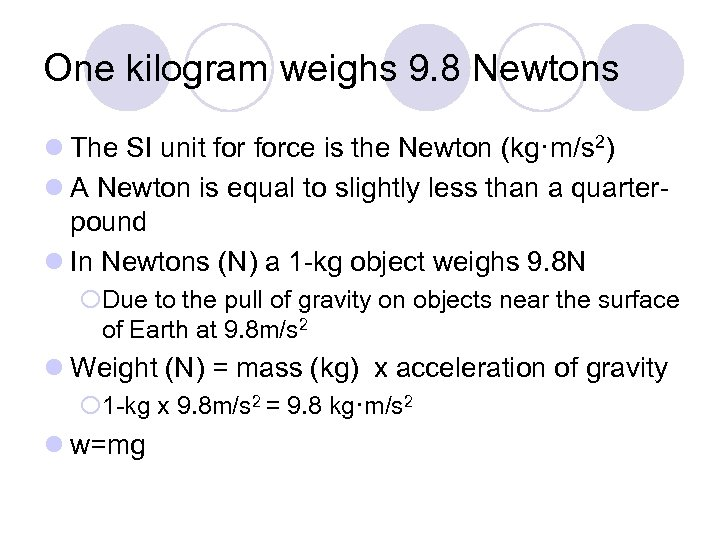One kilogram weighs 9. 8 Newtons l The SI unit force is the Newton (kg·m/s 2) l A Newton is equal to slightly less than a quarterpound l In Newtons (N) a 1 -kg object weighs 9. 8 N ¡Due to the pull of gravity on objects near the surface of Earth at 9. 8 m/s 2 l Weight (N) = mass (kg) x acceleration of gravity ¡ 1 -kg x 9. 8 m/s 2 = 9. 8 kg·m/s 2 l w=mg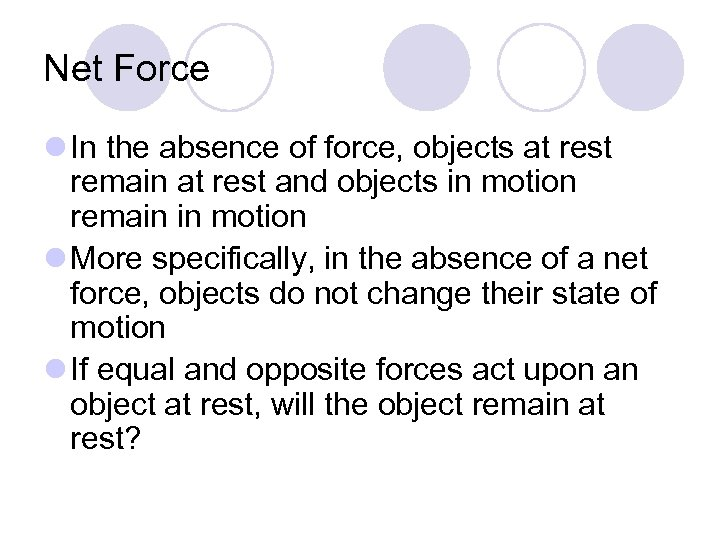Net Force l In the absence of force, objects at rest remain at rest and objects in motion remain in motion l More specifically, in the absence of a net force, objects do not change their state of motion l If equal and opposite forces act upon an object at rest, will the object remain at rest?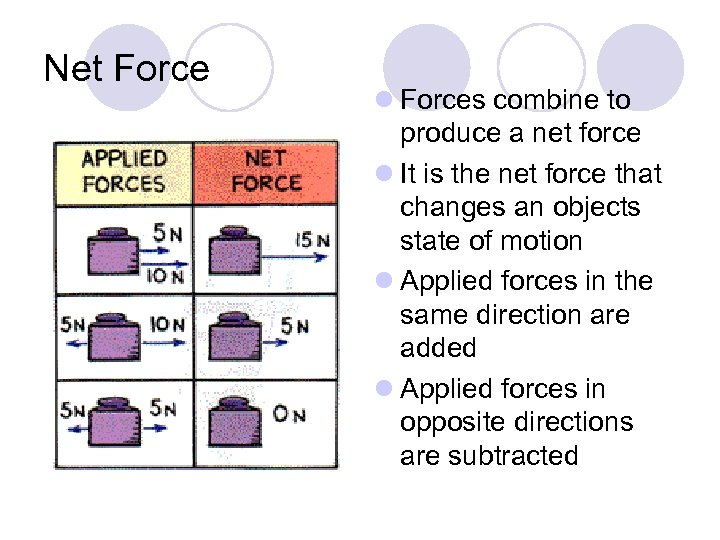Net Force l Forces combine to produce a net force l It is the net force that changes an objects state of motion l Applied forces in the same direction are added l Applied forces in opposite directions are subtracted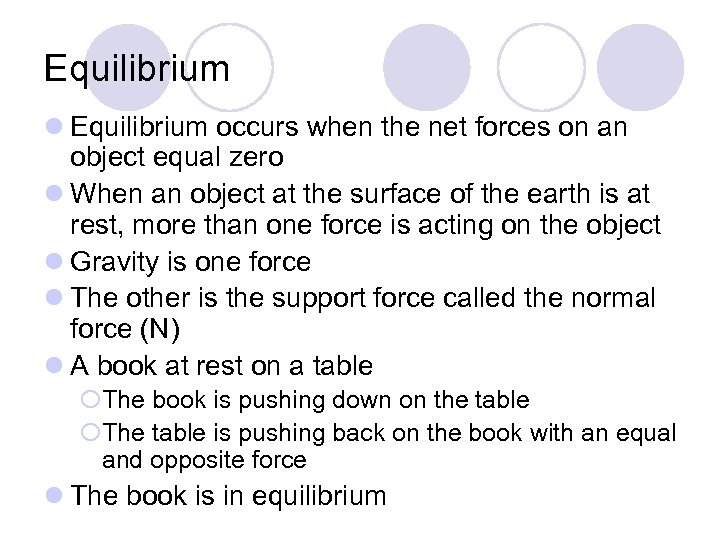Equilibrium l Equilibrium occurs when the net forces on an object equal zero l When an object at the surface of the earth is at rest, more than one force is acting on the object l Gravity is one force l The other is the support force called the normal force (N) l A book at rest on a table ¡The book is pushing down on the table ¡The table is pushing back on the book with an equal and opposite force l The book is in equilibrium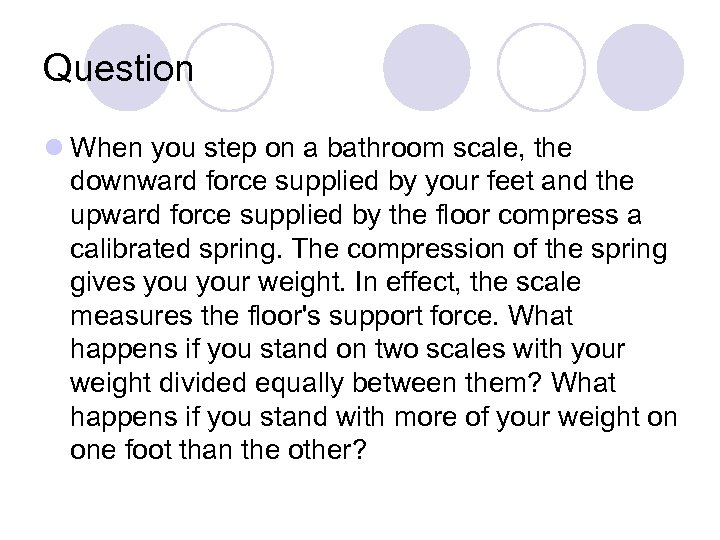Question l When you step on a bathroom scale, the downward force supplied by your feet and the upward force supplied by the floor compress a calibrated spring. The compression of the spring gives your weight. In effect, the scale measures the floor's support force. What happens if you stand on two scales with your weight divided equally between them? What happens if you stand with more of your weight on one foot than the other?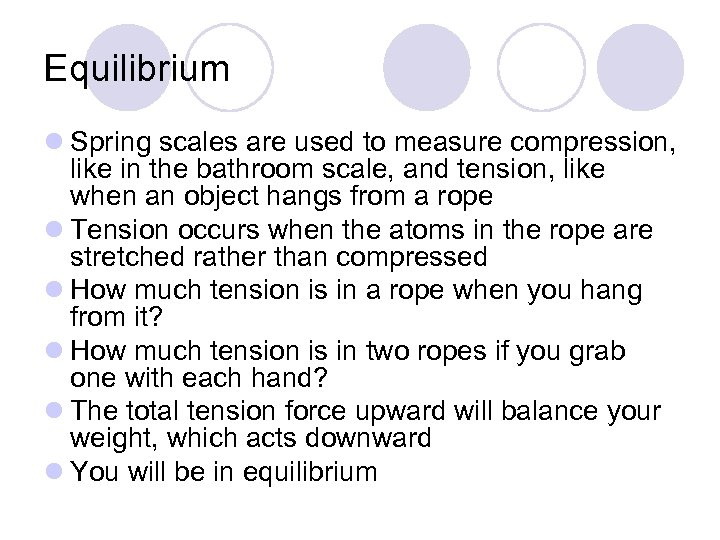Equilibrium l Spring scales are used to measure compression, like in the bathroom scale, and tension, like when an object hangs from a rope l Tension occurs when the atoms in the rope are stretched rather than compressed l How much tension is in a rope when you hang from it? l How much tension is in two ropes if you grab one with each hand? l The total tension force upward will balance your weight, which acts downward l You will be in equilibrium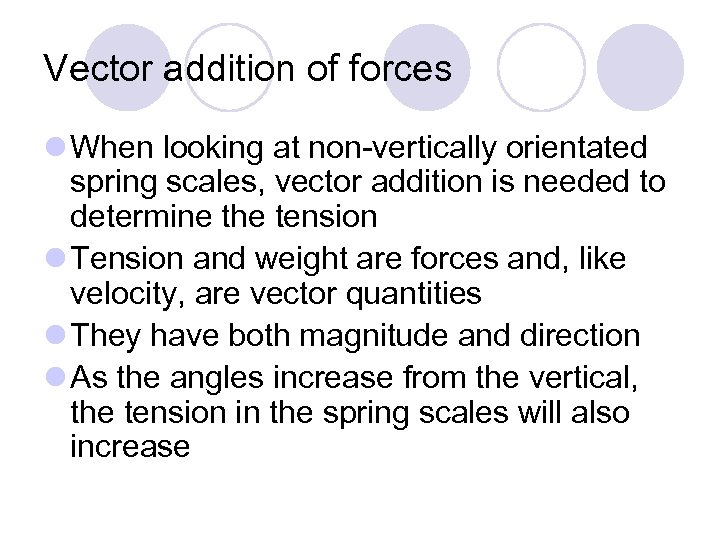Vector addition of forces l When looking at non-vertically orientated spring scales, vector addition is needed to determine the tension l Tension and weight are forces and, like velocity, are vector quantities l They have both magnitude and direction l As the angles increase from the vertical, the tension in the spring scales will also increase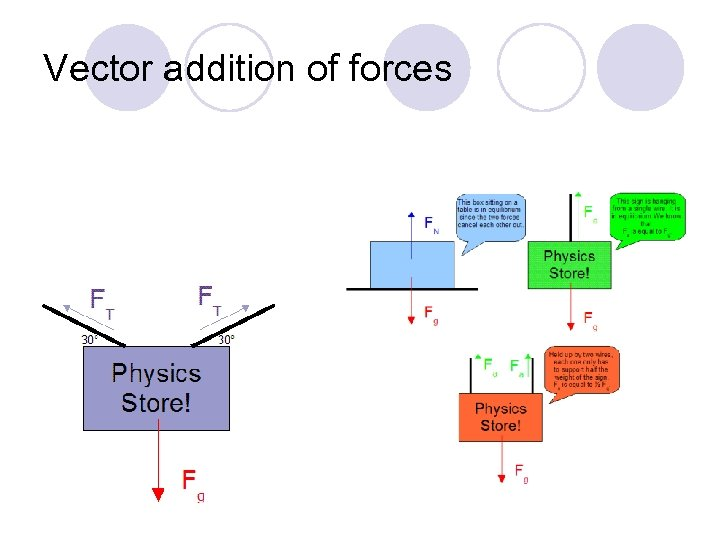Vector addition of forces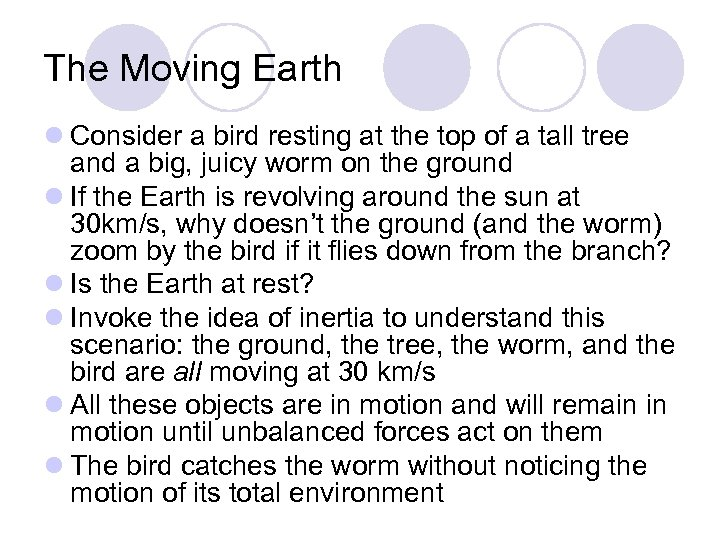The Moving Earth l Consider a bird resting at the top of a tall tree and a big, juicy worm on the ground l If the Earth is revolving around the sun at 30 km/s, why doesn’t the ground (and the worm) zoom by the bird if it flies down from the branch? l Is the Earth at rest? l Invoke the idea of inertia to understand this scenario: the ground, the tree, the worm, and the bird are all moving at 30 km/s l All these objects are in motion and will remain in motion until unbalanced forces act on them l The bird catches the worm without noticing the motion of its total environment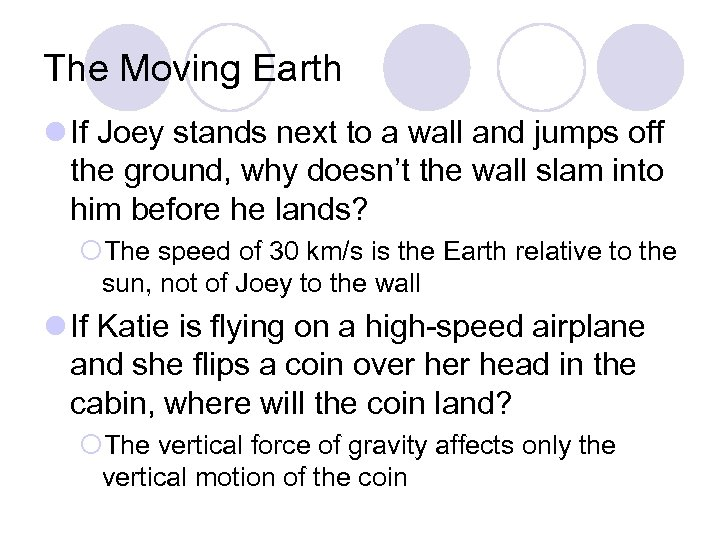The Moving Earth l If Joey stands next to a wall and jumps off the ground, why doesn’t the wall slam into him before he lands? ¡The speed of 30 km/s is the Earth relative to the sun, not of Joey to the wall l If Katie is flying on a high-speed airplane and she flips a coin over head in the cabin, where will the coin land? ¡The vertical force of gravity affects only the vertical motion of the coin